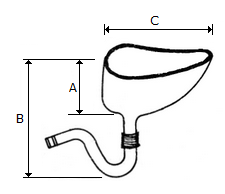# Civil Engineering - Estimating and Costing

### Exercise :: Estimating and Costing - Section 1

26.

In the mid-section formula

 A. The mean depth is the average of depths of two consecutive sections B. The area of mid-sections is calculated by using mean depth C. The volume of the earth work is calculated by multiplying the mid-section area by the distance between the two sections D. The volume of the earth work is calculated by multiplying the mid-section area by the distance between the two original sections E. (a), (b) and (c) of the above.

Explanation:

No answer description available for this question. Let us discuss.

27.

The item of steel work which is measured in sq.m, is

 A. Collapsible gates B. Rolling shutters C. Steel doors D. Ventilators and glazing. E. All the above.

Explanation:

No answer description available for this question. Let us discuss.

28.

The value of 'C' of Indian type W.C. shown in the given figure is :A. 400 mm B. 450 mm C. 500 mm D. 550 mm

Explanation:

No answer description available for this question. Let us discuss.

29.

The area of a sloping surface of a protective embankment of mean height d, side slopes S : 1 and length L is

 A. d x d x s B. d2 x (ds)2 C. L.D 1 + s2 D. 2 LD 1 + s2

Explanation:

No answer description available for this question. Let us discuss.

30.

If the formation level of a highway has a uniform gradient for a particular length, and the ground is also having a longitudinal slope, the earthwork may be calculated by

 A. Mid-section formula B. Trapezoidal formula C. Prismoidal formula D. All the above.